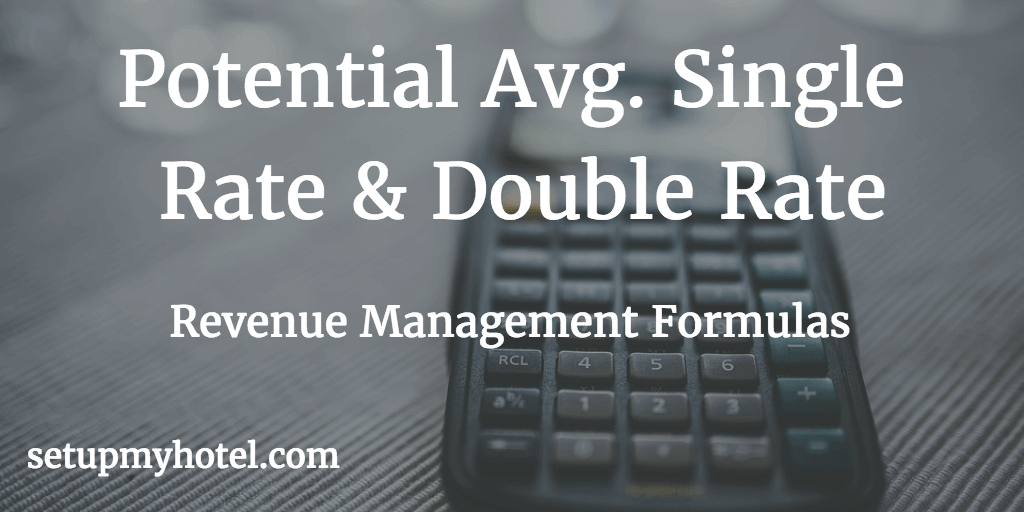# How to Calculate Potential Average Rate Single and Double Rate

One of the main computation involved in the front office or revenue management is to calculate the hotel's yield statistics. Potential Average Rate provides the amount of revenue that would have been generated if all the rooms were sold at their published or rack rate.

For hotels where their single rate and double rate for all room types are same then the PAR for Single & Double will be same as their Rack rate. But in most of the hotels the single rate changes as per room category or type. In such cases an average single rack rate to be calculated.

The Formula for Potential Average Single Rate

Potential Average Single Rate = Single Room Revenue at Published Tariff or Rack Rate / Number of Rooms sold as singles

Potential Avg. Single or Double Rate Calculator

Single Room Rev. or Double Room Rev. at Rack Rate:

Total Single Rooms or Double Rooms Sold:

Potential Avg. Single Rate or Double Rate:

Example 1 - Potential Avg. Single Rate (Where the single rate is same for all room types):

Total Number of Rooms sold in Single     = 25

Rack Rate / Published Tariff for Single     = 125.00

Single Room Revenue at Published Tariff = 25 * 125.00

= 3125.00

Potential Avg. Single Rate  for 01st Jan 2017  = 3125.00 / 25

= 125.00

Example 2 - Potential Avg. Single Rate (Where the single rate varies as per room types):

Deluxe Room - Rack Rate / Published Tariff for Single    = 125.00

Deluxe Room Number of Rooms Available in the hotel   = 100

= 125.00 * 100

= 12500.00

Suite Room - Rack Rate / Published Tariff for Single      = 168.00

Suite Room Number of Rooms Available in the hotel      = 30

= 168.00 * 30

= 5040.00

Single Room Revenue at Published Tariff = (12500.00 + 5040.00)

= 17540.00

Potential Avg. Single Rate  for 01st Jan 2017 = 17540.00 / 130

= 134.92

Formula for Potential Average Double Rate

Potential Average Double Rate = Double Room Revenue at Published Tariff or Rack Rate / Number of Rooms sold as Double

Example 1 - Potential Avg. Double Rate (Where the Double rate is same for all room types):

Total Number of Rooms sold in Double    = 55

Rack Rate / Published Tariff for Double    = 175.00

Double Room Revenue at Published Tariff = 55 * 175.00

= 9625.00

Potential Avg. Double Rate  for 01st Jan 2017  = 9625.00 / 55

= 175.00

Example 2 - Potential Avg. Double Rate (Where the Double rate varies as per room types):

Deluxe Room - Rack Rate / Published Tariff for Double  = 185.00

Deluxe Room Number of Rooms Available in the hotel   = 100

= 185.00 * 100

= 18500.00

Suite Room - Rack Rate / Published Tariff for Double     = 215.00

Suite Room Number of Rooms Available in the hotel      = 30

= 215.00 * 30

= 6450.00

Double Room Revenue at Published Tariff = (18500.00 + 6450.00)

= 24950.00

Potential Avg. Double Rate  for 01st Jan 2017 = 17540.00 / 130

= 134.92